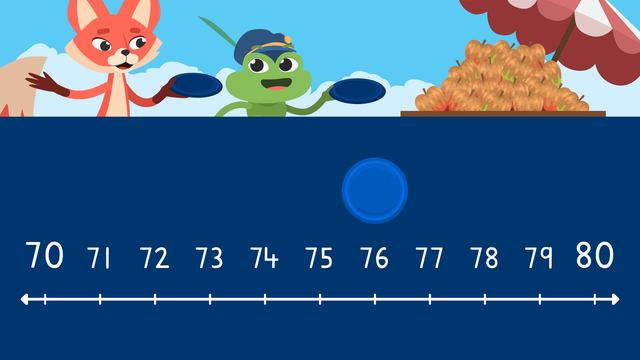# Rounding using a number line (tens)Rate this video

Ø 5.0 / 2 ratings

The authorTeam Digital
Rounding using a number line (tens)
CCSS.MATH.CONTENT.3.NBT.A.1

## Information about the videoRounding using a number line (tens)

### Rounding to the Nearest 10 on a Number Line

This video shows how to round to the nearest ten on a number line. How to set up up a number line for rounding, and when to round up or round down is covered in the video. There are also rounding to the nearest ten on a number line worksheets below for further practice.

### TranscriptRounding using a number line (tens)

Skylar and Henry are participating in a frisbee competition, where the top three finishers will receive all you can carry caramel apples! They each get one throw. The spot where the frisbee lands is rounded to the nearest ten, and this becomes their score. Skylar steps up for her throw. Now it is Henry's turn. Their fierce competitor, Benji, throws last. To calculate their scores we can use rounding to the nearest ten on a number line. When rounding to the nearest ten on a number line, first identify the number you are rounding. Next, set up the lesser tens value on the left side and the higher tens value on the right side. Then fill in all the numbers between the tens. The number line is ready, so we plot the number we are rounding on it. If the number is below halfway, we round down to the nearest ten, and if the number is halfway or above, we round up to the nearest ten. Let's practice with Benji's throw. Here is where Benji's throw landed. First identify the number we are using, which is forty-five. The lesser tens value is forty, so write it at the beginning, and the higher tens value is fifty, so write it at the end. Now fill in the number line with all the numbers between forty and fifty. Benji scored forty-five, so we can plot it here on the number line. We can see that forty-five is at the halfway point on the number line, so we round up to fifty. Forty-five rounded to the nearest ten is fifty. Let's see if you can help calculate Skylar and Henry's score! Here is where Skylar's frisbee landed. Can you identify the number? It is seventy-six. What is the lesser tens value and higher tens value of seventy-six? The lesser tens value is seventy, and the higher tens value is eighty. Now we fill in all the numbers between seventy and eighty. Can you remember the next step? We plot Skylar's frisbee throw, so seventy-six goes here. Now we need to decide if we round up, or round down. Which should we do for Skylar's score? Seventy-six is over halfway on the number line, so we round up to the nearest ten. Seventy-six rounded to the nearest ten is eighty. Now let's calculate Henry's score. Here is where Henry's frisbee landed. What is the number? It is sixty-four. What is the lesser tens value and higher tens value of sixty-four? The lesser tens value is sixty and the higher tens value is seventy. Now we fill in all the numbers between sixty and seventy. What is the next step? We plot Henry's frisbee throw, so sixty-four goes here. Now we need to decide if we round up, or round down. Which should we choose for Henry's score? Sixty-four is below halfway on the number line, so we round down to the nearest ten. Sixty-four rounded to the nearest ten is sixty. Remember, to round to the nearest ten on a number line, first identify the number you are rounding. Next, set up the lesser tens value on the left side and the higher tens value on the right side. Then fill in all the numbers between the tens. Now the number line is ready, we plot the number we are rounding on the number line. If the number is below halfway we round down to the nearest ten, and if the number is halfway or above we round up to the nearest ten. Well, it looks like Skylar was the overall winner. Great job, Skylar. But don't forget, everyone in the top three gets all you can carry caramel apples. Wow, Benji and Henry, you both sure have a lot of caramel apples there! Of course, Skylar, cunning as always.